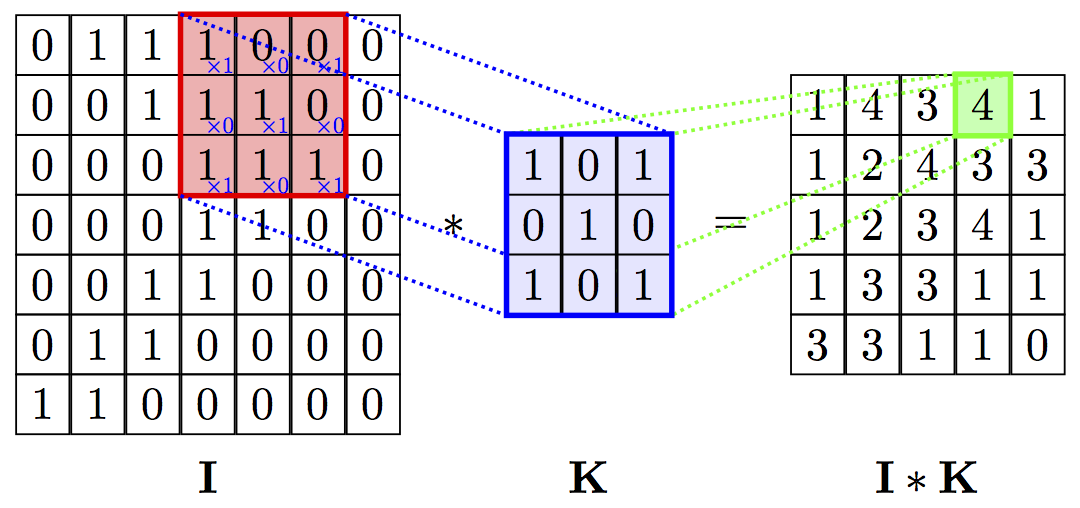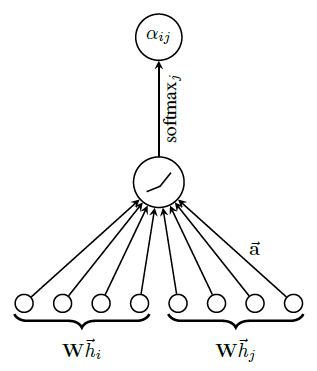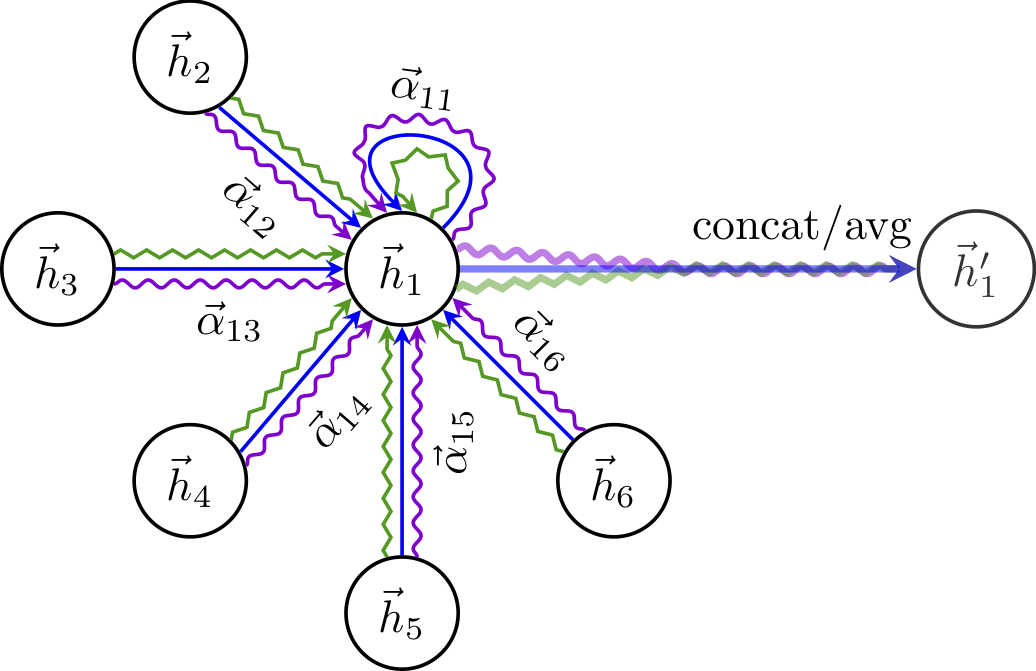ICLR18一篇解决GCN聚合信息时无法区分信息重要性的论文

### 做法及创新

#### 图卷积#### Self-attention

1. 基于顶点的特征计算系数$e_{ij}$
1. 以顶点的邻域将上一步计算得到的系数正则化，这么做能引入图的结构信息：DeepInf中给出了证明：

​ 将权重向量$\overrightarrow{a}\in \mathbb{R}^{2F’}$重写为$\overrightarrow{a}=[p^T，q^T]$，则有

​ 由softmax与LeakyReLU的单调性可知，因为$a_{ij}>a_{ik}$，有$q^TWh_j>q^TWh_k$，类似地就可以得到$a_{i’j}>a_{i’k}$。

​ 这意味着，即使每个顶点都只关注于自己的邻域，但得到的attention系数却具有全局性。

1. 以上一步得到的系数$\alpha_{ij}$作为顶点$j$的特征对顶点$i$的重要程度，将领域中各顶点的特征做一个线性组合以作为顶点$i$最终输出的特征表示：